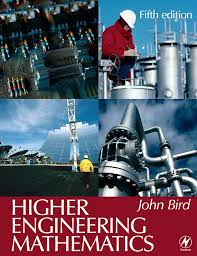Wednesday, July 29, 2015

Higher Engineering Mathematics by John BirdJohn Bird’s approach, based on numerous worked examples and interactive problems, is ideal for students from a wide range of academic backgrounds, and can be worked through at the student’s own pace. Basic mathematical theories are explained in a straightforward manner, being supported by practical engineering examples and applications in order to ensure that readers can relate theory to practice. The extensive and thorough topic coverage makes this an ideal text for a range of university degree modules, foundation degrees, and HNC/D units.

Now in its sixth edition, Higher Engineering Mathematics is an established textbook that has helped many thousands of students to gain exam success. It has been updated to maximise the book’s suitability for first year engineering degree students and those following foundation degrees. This book also caters specifically for the engineering mathematics units of the Higher National Engineering schemes from Edexcel. As such it includes the core unit, Analytical Methods for Engineers, and two specialist units, Further Analytical Methods for Engineers and Engineering Mathematics, both of which are common to the electrical/electronic engineering and mechanical engineering pathways. For ease of reference a mapping grid is included that shows precisely which topics are required for the learning outcomes of each unit.